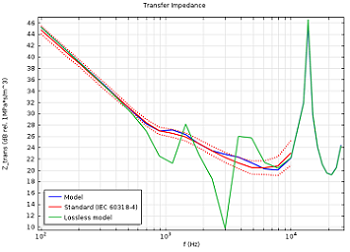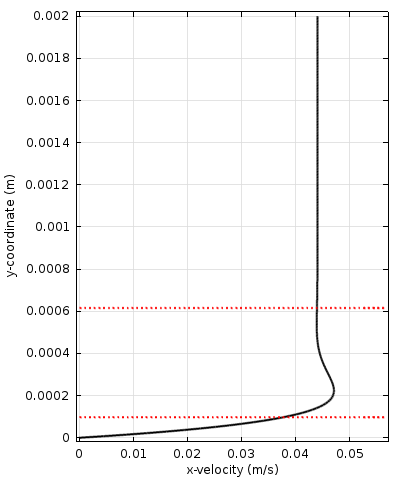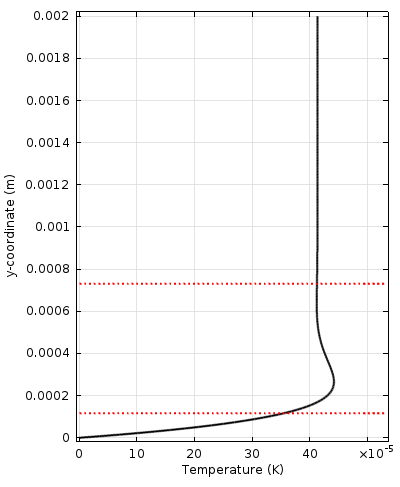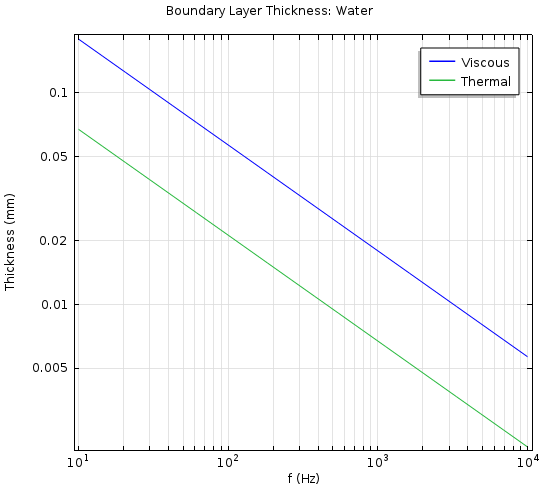# 热粘性声学的理论基础：热损耗与粘性损耗

2014年 2月 27日

### 热粘性声学简介### 探究热粘性声学背后的物理场

#### 推导控制方程

p = p_0+p'e^{i\omega t}, \quad \mathbf{u} = \mathbf{u}_0+\mathbf{u}' e^{i\omega t}, \quad T = T_0 + T' e^{i\omega t}

i\omega\rho =-\rho_0 (\nabla\cdot\mathbf{u})

i\omega\rho_0 \mathbf{u} = \nabla\cdot \left(-p\mathbf{I}+\mu ( \nabla\mathbf{u}+(\nabla\mathbf{u})^T )+\left(\mu_\textrm{B}-\frac{2}{3}\mu \right)(\nabla\cdot\mathbf{u})\mathbf{I} \right)

i\omega (\rho_0 C_p T -\ T_0 \alpha_0 p) = -\nabla\cdot(-\textrm{k}\nabla T) + Q

\rho = \rho_0 (\beta_T p -\alpha_0 T)

#### 粘性边界层与热边界层\textrm{Pr} = \frac{C_p \mu}{\textrm{k}} \qquad \delta_\textrm{visc} = \sqrt{\textrm{Pr}} \: \delta_\textrm{therm}

\delta_\textrm{visc} = \sqrt{\frac{2\mu}{\omega\rho_0}} \qquad \delta_\textrm{therm} = \sqrt{\frac{2 \textrm{k}}{\omega\rho_0 C_p}}#### 材料参数

• 动力粘度，\mu
• 动力粘度 \mu 用来度量流体内部抵抗剪切力的阻力的大小。动力粘度是常数，定义为应力与应变速率之比。动力粘度与运动粘度 \nu 的关系为 \mu = \rho_0 \: \nu。动力粘度有时也用符号 \eta 来表示。
• 容积粘度，\mu_\textrm{B}
• 容积粘度又称体积粘性系数、第二粘性系数或膨胀粘性系数。它与因流体的膨胀和压缩而产生的损耗有关。\mu_\textrm{B} 出现在应力张量项中（方程 3 的右侧），该项与体相流的压缩率（\nabla\cdot\mathbf{u}）存在关联。这一因素难以测量，往往取决于频率的高低。
• 恒压热容（比热容），C_p
• 恒压热容这一材料参数用来描述流体温度变化所需的热量（恒压条件下）。
• 导热系数，\textrm{k}
• 导热系数是 Fourier 热传导定律中，温度梯度与热通量之间的比例系数。
• 热膨胀系数（等压），\alpha_0
• 热膨胀系数用于描述流体在热作用下的体积扩大现象，反映了温度升高时流体的膨胀能力。
• 等温压缩率，\beta_T
• 等温压缩率是流体状态方程的一个重要参数，用流体内的压力变化与体积变化之比进行表示。等温压缩率与常规（等熵）压缩率的关系可以通过比热率表示为 \beta_T = \gamma \beta_s

### 扩展阅读与参考文献

• The “Thermoviscous Acoustics Branch” section in the Acoustics Module User’s Guide of the COMSOL Documentation
• D. T. Blackstock, “Fundamentals of Physical Acoustics”, John Wiley and Sons, 2000
• S. Temkin, “Elements of Acoustics”, Acoustical Society of America, 2001
• B. Lautrup, “Physics of Continuous Matter”, Second Edition, CRC Press, 2011
• P. M. Morse and K. U. Ingard, “Theoretical Acoustics”, Princeton University Press
• A. D. Pierce, “Acoustics: An Introduction to Its Physical Principles and Applications”, Acoustical Society of America, 1989
• A. S. Dukhin and P. J. Goetz, “Bulk viscosity and compressibility measurements using acoustic spectroscopy”, J. Chem. Phys. 130, 124519 (2009)